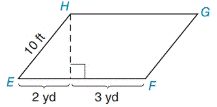Chapter 8.1, Problem 14EElementary Geometry For College St...

7th Edition
Alexander + 2 others
ISBN: 9781337614085

Solutions

Chapter
SectionElementary Geometry For College St...

7th Edition
Alexander + 2 others
ISBN: 9781337614085
Textbook Problem

In Exercises 9 to 18, find the areas of the figures shown or described.▱ E F G H

To determine

To find:

The area of EFGH

Explanation

1) Approach:

Area of the parallelogram is given by,

A=bh unit2.

Where b is the base of the length, h is the height.

2) Calculation:

Given,

In the parallelogram EFGH with side (s)=10 ft.

base (b)=(2+3)yd.

Let point I that lies on EF

Then HI¯ is the height of the parallelogram EFGH.

Find length of height (h):

For right triangle EIH,

Since, 1 yd=3 ft

EI=2 yd=6 ft

EH=10 ft

HI=h ft

By the Pythagorean theorem,

(EI)2+(HI)2=(EH)2

(6)2+(h)2

Still sussing out bartleby?

Check out a sample textbook solution.

See a sample solution

The Solution to Your Study Problems

Bartleby provides explanations to thousands of textbook problems written by our experts, many with advanced degrees!

Get Started

In Exercises 4562, find the values of x that satisfy the inequality (inequalities). 55. (x + 3)(x 5) 0

Applied Calculus for the Managerial, Life, and Social Sciences: A Brief Approach

In Exercises 1-4, simplify the expression. 2x22x124x224x+36

Calculus: An Applied Approach (MindTap Course List)

Use the matrices below a needed to complete Problems 1-25, Write the negative of matrix

Mathematical Applications for the Management, Life, and Social Sciences

Solve the equations in Exercises 112 for x (mentally, if possible). x3=1

Finite Mathematics and Applied Calculus (MindTap Course List)

12,554+22,606+11,460+20,005+4,303=

Contemporary Mathematics for Business & Consumers

Multiply. (x1)2

Trigonometry (MindTap Course List)

True or False: n=1(1)nnn+3 converges.

Study Guide for Stewart's Single Variable Calculus: Early Transcendentals, 8th